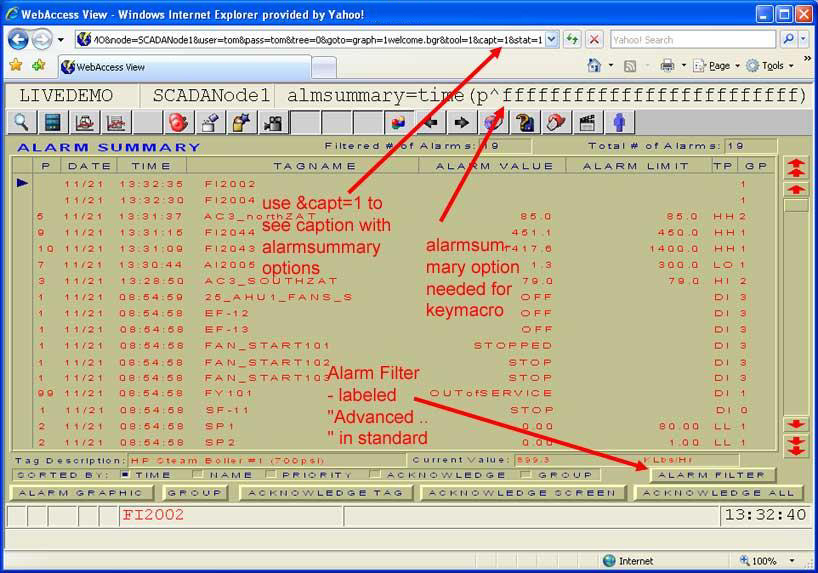11.1.42    <GOTO>ALMSUMMARY

Description:   Changes display in current window to the alarm summary of active alarms.

Syntax:         <GOTO>ALMSUMMARY

Arguments:    ALMSUMMARY
ALMSUMMARY=time(p^c000000000000000000000000)
ALMSUMMARY=priority(p^a000000000000000000000000)
ALMSUMMARY=priority(p^e0000ffffffffffffffffffff)
ALMSUMMARY=name(p^9000000000000000000000000)
ALMSUMMARY=time(ack^p^8800000000000000000000000)
ALMSUMMARY=time(unack^p^8400000000000000000000000)
ALMSUMMARY=time(remote^ack^p^8200000000000000000000000)
ALMSUMMARY=time(local^unack^p^8100000000000000000000000)

Examples:      <GOTO>ALMSUMMARY

The keymacro Shortcut is <F5> and is the same as the F5 function key on the keyboard.

To determine the options to filter alarms, use the ADVANCED button in the alarm summary (sometimes labelled ALARM FILTER) and view the caption bar in VIEW.